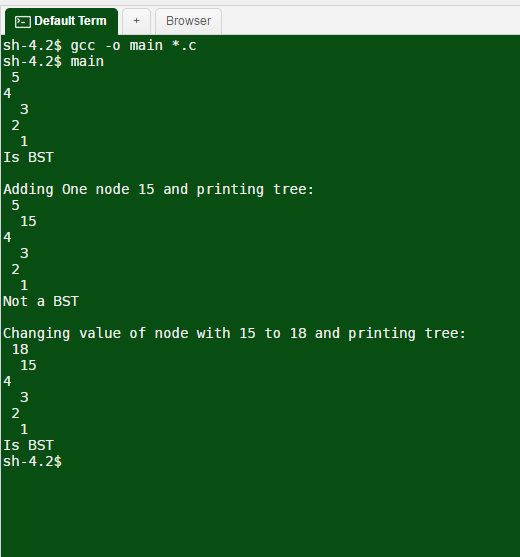# Answered! Write an algorithm that a given Binary tree is a binary search tree. NOTE: please submit code with at least three (3) test cases in C++…

Write an algorithm that a given Binary tree is a binary search tree.

NOTE: please submit code with at least three (3) test cases in C++

Don't use plagiarized sources. Get Your Custom Essay on
Answered! Write an algorithm that a given Binary tree is a binary search tree. NOTE: please submit code with at least three (3) test cases in C++…
GET AN ESSAY WRITTEN FOR YOU FROM AS LOW AS \$13/PAGE

#include <iostream>

#include <limits.h>
#include <stdlib.h>
#include <iomanip>

using namespace std;

/* Struct to declare the node for Binary tree */
struct node
{
int data;
struct node* left;
struct node* right;
};

/* Recursive function to check if the tree is BST */
int isSearchTreeUtil(struct node* root, int min, int max)
{
/* If node is empty, its a valid tree */
if (root==NULL)
return 1;

/* If the data of the root is not under the min/max constraints */
if (root->data < min || root->data > max)
return 0;

/*
This node is fine, recursively check for left and right half, and then return result
Left half will have values lesser while right half will have larger values as compared
to the root node
*/
return
isSearchTreeUtil(root->left, min, root->data-1) &&
isSearchTreeUtil(root->right, root->data+1, max);
}

/* Check if Binary Tree is a binary search tree */
int isSearchTree(struct node* node)
{
// The minimum in the tree can be -infinity, and max can be +infinity
// we define infinity here using INT_MIN and INT_MAX
return(isSearchTreeUtil(node, INT_MIN, INT_MAX));
}

/* Function to create new tree node */
struct node* createNode(int data)
{
struct node* node = (struct node*) malloc(sizeof(struct node));
node->data = data;
node->left = NULL;
node->right = NULL;

return(node);
}

void printTree(struct node* root, int space)
{
// Base case
if (root == NULL)
return;

// Increase distance between levels
space += 1;

// Process right child first
printTree(root->right, space);

// Print current node after space
// count
int i;
for ( i = 1; i < space; i++)
cout << ” “;
cout << root->data << endl;

// Process left child
printTree(root->left, space);
}

/* Driver program to test*/
int main()
{
// Create a tree
struct node *root = createNode(4);
root->left = createNode(2);
root->right = createNode(5);
root->left->left = createNode(1);
root->left->right = createNode(3);

printTree(root, 0);

if(isSearchTree(root))
cout << “Is BST” << endl;
else
cout << “Not a BST” << endl;

cout << “nAdding One node 15 and printing tree: n”;
root->right->left = createNode(15);
printTree(root, 0);
if(isSearchTree(root))
cout << “Is BST” << endl;
else
cout << “Not a BST” << endl;

cout << “nChanging value of node with 15 to 18 and printing tree: n”;
root->right->data = 18;
printTree(root, 0);
if(isSearchTree(root))
cout << “Is BST” << endl;
else
cout << “Not a BST” << endl;

return 0;
}